﻿ 土地价格市场化对中国工业部门要素投入与技术选择的影响
 财经研究2016, Vol. 42Issue (8): 85-960

#### 文章信息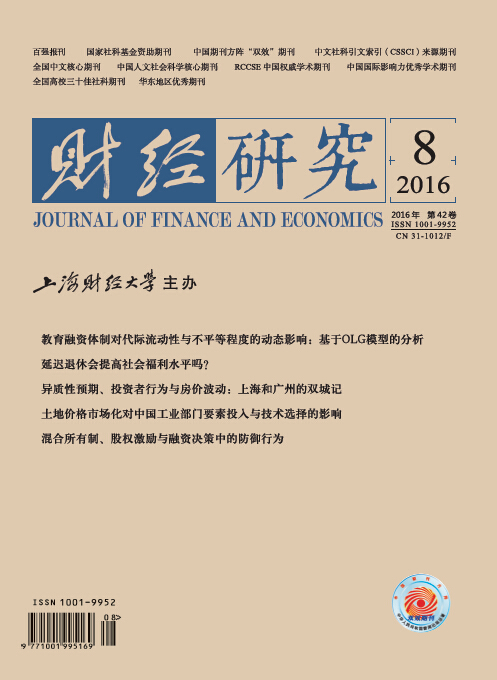财经研究2016年42卷第8期

Zhao Aidong, Lan Jing, Ma Xianlei, Xu Shi.

The Impact of Land Price Marketization on Factor Input and Technology Choice in China's Industrial Sector

Journal of Finance and Economics, 2016, 42(8): 85-96.

### 文章历史《财经研究》
2016第42卷第8期

1.南京农业大学公共管理学院，江苏 南京 210095;
2.中国土地勘测规划院，北京 100035

The Impact of Land Price Marketization on Factor Input and Technology Choice in China's Industrial Sector
Zhao Aidong1, Lan Jing1, Ma Xianlei1, Xu Shi2
1.School of Public Administration, Nanjing Agriculture University, Nanjing 210095, China;
2.China Land Surveying and Planning Institute, Beijing 100035, China
Abstract: Land price marketization is a key component of factor price reform in China. Based on panel data in industrial sector from 2007 to 2013, this paper empirically analyzes the impact of relative price changes of industrial land on factor input adjustment and biased technical progress in China's industrial sector. It comes to the results as follows:in the short run, the rise in relative prices of industrial land suppresses the demand of industrial land, and induces the industrial sector to increase the relative input proportion of other factors to reduce the amount of industrial land in East China, while at the national level and in Central China & West China, short-term price-induced substitution effect of relative price changes of industrial land does not hold; in the long run, the increase in indusrial land prices leads to the use of energy & material capital input-saving and intellectual capital input-using technological progress; and the land input-saving technological progress feature is only significantly supported in East China, and is not obvious at the national level and in Central China and West China, which is closely related to the marketization reform of industrial land prices oriented by Chinese governments. Therefore, in the future China should regard the land price marketization reform as an opportunity, pay full attention to the incentive effect of land price lever on fator structure optimization and technological innovation in industrial sector, to promote the transformation of industrial growth pattern.
Key words: industrial land    price marketization    price-induced technological progress    factor substitution图 1 中国工业用地出让方式结构(2007-2013年)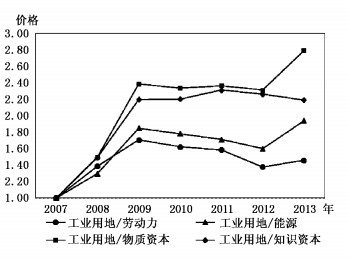图 2 工业用地相对价格走势(2007-2013年)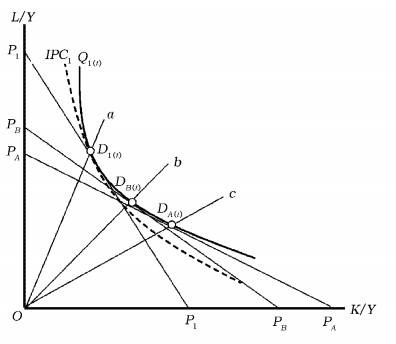图 3 工业用地价格与短期要素投入变动图 4 工业用地价格与长期技术选择
(一) 工业用地价格变动与短期要素投入变动

H1：短期内，工业用地相对价格上升会诱致工业部门使用其他要素替代工业用地，工业用地相对投入比例会出现下降，这种短期诱致替代效应在发达地区更加显著。

(二) 工业用地价格变动与长期技术选择

H2：长期内，工业用地相对价格上升会诱致工业部门采用土地节约型技术进步化解成本上升压力，这种长期技术创新诱致效应在发达地区更加显著。

 $C=G\left({y, p, \rho, X, T} \right)$ (1)

(1)式中G(·)为长期规模报酬不变的成本函数，并且对于要素价格是线性齐次、非递减的凹函数，对于产出y是非递减的。y代表产出，P是要素X的当前市场价格，ρ是依赖于要素当前和过去价格的要素长期价格。X为投入要素，包含劳动力W、能源E、工业用地L、物质资本Kw和知识资本KeT代表企业的技术状态并可分为两部分：第一部分表示与企业R & D投入和要素价格变动无关的外生技术，即时间趋势变量t；第二部分是与要素价格变动相关的企业内生技术，本文称之为价格诱致性技术进步。

 $\begin{array}{l} {C_t}={\alpha _0} + {\alpha _y}{\rm{ln}}{y_t} + \sum\limits_{i=1}^5 {{\beta _i}{\rm{ln}}{P_{it}}} + {\alpha _t}t + \frac{1}{2}\left({{\gamma _{yy}}{\rm{l}}{{\rm{n}}^{\rm{2}}}{y_t} + \sum\limits_{i=1}^5 {\sum\limits_{j=1}^5 {{\beta _{ij}}{\rm{ln}}{P_{it}}{\rm{ln}}{P_{jt}} + {\alpha _{tt}}{t^2}} } } \right)\\ \; \;\; \;\; \;\; + \sum\limits_{i=1}^5 {{\beta _{it}}{\rm{ln}}{P_{it}}t} + \sum\limits_{i=1}^5 {{\beta _{iy}}{\rm{ln}}{P_{it}}{\rm{ln}}{y_t}} + {\gamma _{yt}}t{\rm{ln}}{y_t} \end{array}$ (2)

(2)式中，i，j＝W，E，L，Kw，Ke。(1)式中的外生技术进步在(2)式中表示为时间趋势t，而价格诱致性技术进步的发生涉及企业对未来要素价格变化的预期，只有预期要素相对价格在较长一段时期内持续失衡时，企业才会加大投入进行生产技术创新。价格诱致的技术动态调整存在滞后期，这种诱致机制的发生依赖于R & D投入等科技创新资源对要素长期价格的响应。因此价格诱致机制可以看成要素长期价格ρ的某种函数形式。参照Esposti和Pierani(2008)等人的研究，本文根据考伊克几何分布滞后模型建立价格诱致的动态过程，即：

 ${\rho _{it}}=\lambda {p_{i, t-1}} + {\lambda ^2}{p_{i, t-2}} +... + {\lambda ^\tau }{p_{i, t-\tau-1}}=\sum\limits_{\tau=0}^\infty {{\lambda ^\tau }} {p_{i, t-\tau-1}}=\frac{1}{{1-\lambda }}{p_{i, t-1}}\left({1 < \lambda < 1} \right)$ (3)

(3)式隐含着当期价格的诱致效应从t-1期开始随着滞后期的增加而几何衰减，而λ决定了滞后衰减的速度，λ越接近0，衰减速度越快，λ越大则表示价格的诱致效应越大。

 ${S_{it}}{\rm{=}}{X_{it}} \cdot \frac{{{P_{it}}}}{C}{\rm{=}}\frac{{\partial {\rm{ln}}C}}{{\partial {\rm{ln}}{P_{it}}}}=\frac{{\partial C}}{{\partial {P_{it}}}} \cdot \frac{{{P_{it}}}}{C}={\beta _i} + \sum\limits_{j=1}^5 {{\beta _{ij}}} {\rm{ln}}{P_{jt}} + t{\beta _{it}} + {\beta _{iy}}{\rm{ln}}{y_t}$ (4)

 ${S_{it}}=\frac{{\partial {\rm{ln}}C}}{{\partial {\rm{ln}}{\rho _{it}}}}=\lambda {S_{it-1}} + (1-\lambda){\beta _i} + \sum\limits_{j=1}^5 {{\beta _{ij}}{\rm{ln}}{P_{jt}}} + {\beta _{it}}t + {\beta _{iy}}{\rm{ln}}{y_t}$ (5)

 $\sum\limits_i {{\beta _i}}=1, \quad \sum\limits_i {{\beta _{iy}}}=0, \quad {\beta _{ij}}={\beta _{ji}}, \quad \sum\limits_i {{\beta _{ij}}}=0, \quad {\beta _{ji}}={\beta _{ij}}$
(二) 要素投入变动和技术选择方程

1.要素替代与要素投入变动方程。要素投入的变动是工业部门在要素相对价格变动的情况下通过要素替代等一系列行为实现的，而Morishima替代弹性可以较准确地估计两种要素投入比率对相对价格的反应程度(黄光晓，2011)。因此，本文用Morishima替代弹性来衡量生产中任意两种生产投入要素的替代程度，公式为：

 ${\sigma _{ij}}=\frac{{\partial {\rm{ln}}({x_i}/{x_j})}}{{\partial {\rm{ln}}{p_j}}}=\frac{{\partial {\rm{ln}}{x_i}}}{{\partial {\rm{ln}}{p_j}}}-\frac{{\partial {\rm{ln}}{x_j}}}{{\partial {\rm{ln}}{p_j}}}={\varepsilon _{ij}}-{\varepsilon _{jj}}$ (6)

 ${\varepsilon _{ij}}=\frac{{\partial ln{x_i}}}{{\partial ln{p_j}}}{\rm{=}}\frac{{\partial ln{S_i}}}{{\partial ln{p_j}}} + \frac{{\partial lnC}}{{\partial ln{p_j}}}-\frac{{\partial ln{p_i}}}{{\partial ln{p_j}}}=\frac{{{\beta _{ij}}}}{{{S_i}}} + {S_j}, 如果i \ne j, 则{\varepsilon _{jj}}=\frac{{\partial ln{x_j}}}{{\partial ln{p_j}}}=\frac{{{\beta _{jj}}}}{{{S_j}}} + {S_j}-1$ (7)

2.要素相对价格变动与技术选择方程。要素相对价格变动不仅导致了要素投入变动，长期也会对部门技术选择产生影响，沿用Peeters和Surry(2000)、Esposti和Pierani(2008)等的做法，定义诱致性技术进步为随着长期价格变动部门生产成本减少的幅度，工业用地价格变动诱致的技术进步可以表示为：

 ${\gamma _{cl}}=\partial {\rm{ln}}C/\partial {\rm{ln}}{\rho _L}=\partial {\rm{ln}}C/(\partial {\rm{ln}}{p_L}/(1-\lambda))=(\partial {\rm{ln}}C/\partial {\rm{ln}}{p_L})/(1-\lambda)$ (8)

γCl < 0，说明工业用地价格上涨在长期内确实诱致部门内部开发或使用新技术来化解成本上升压力。进一步，结合要素投入份额函数(4)，参考Binswanger(1974)、Celikkol(1999)等人关于价格变化诱致的技术进步对要素投入决策的测算方法，诱致性技术进步对要素投入产生的效应表示为：

 ${\psi _{iL}}=\partial {s_i}/\partial {\rm{ln}}{\rho _L}=\partial {s_i}/(\partial {\rm{ln}}{p_L}/(1-\lambda))=({s_i}({\varepsilon _{iL}}-{\gamma _{Cl}}))/(1-\lambda)$ (9)

(9)式中，si表示第i要素在总成本C中所占的份额，εil为工业用地与第i种投入要素的替代弹性，iWEKwKeψil < 0(或ψil>0)表示工业用地价格上涨导致i要素投入份额减少(增加)，说明工业用地价格上涨诱致了i要素节约型(使用型)技术进步。

 ${B_{it}}=\partial {s_i}/\partial t={s_i}({\varepsilon _{it}}-{\varepsilon _{ct}})$ (10)

(三) 估计方法

 $\left\{ \begin{array}{l} {S_W}{\rm{=}}{\lambda _W}{S_{W, t-1}} + \beta _W^* + {\beta _{WW}}{\rm{ln}}P_W^* + {\beta _{WE}}{\rm{ln}}P_E^* + {\beta _{wL}}{\rm{ln}}P_L^*\\ \; \;\; \;\; \;\; + {\beta _{wKw}}{\rm{ln}}P_{{K_w}}^* + {\beta _{wt}}t + {\beta _{wy}}{\rm{ln}}{y_t} + {\varepsilon _W}\\ {S_E}{\rm{=}}{\lambda _E}{S_{E, t-1}} + \beta _E^* + {\beta _{EE}}\ln P_E^* + {\beta _{EW}}{\rm{ln}}P_W^* + {\beta _{EL}}{\rm{ln}}P_L^*\\ \; \;\; \;\; \;\; + {\beta _{EK{\rm{w}}}}{\rm{ln}}P_{{K_W}}^* + {\beta _{Et}}t + {\beta _{Ey}}{\rm{ln}}{y_t} + {\varepsilon _E}\\ {S_L}{\rm{=}}{\lambda _L}{S_{L, t-1}} + \beta _L^* + {\beta _{LL}}{\rm{ln}}P_L^* + {\beta _{LW}}{\rm{ln}}P_W^* + {\beta _{LE}}{\rm{ln}}P_E^*\\ \; \;\; \;\; + {\beta _{LKw}}{\rm{ln}}P_{Kw}^* + {\beta _{Lt}}t + {\beta _{Ly}}{\rm{ln}}{y_t} + {\varepsilon _L}\\ {S_K}_w{\rm{=}}{\lambda _{Kw}}{S_K}_{w, t-1} + \beta _{K{\rm{w}}}^* + {\beta _{KwKw}}{\rm{ln}}P_{Kw}^* + {\beta _{KwW}}\ln P_W^*\\ \; \;\; \;\; \; + {\beta _{KwL}}\ln P_L^* + {\beta _{KwE}}\ln P_E^* + {\beta _{Kwt}}t + {\beta _{Kwy}}{\rm{ln}}{y_t} + {\varepsilon _{Kw}} \end{array} \right.$ (11)

1.产出(y)。选用工业总产值衡量。

2.物质资本投入(Kw)和资本价格(PKw)。采用工业行业资本存量作为资本投入，资本存量使用永续盘存法计算(张军，2004)。关于资本价格，一般认为资本租赁价格可作为资本真实使用成本，本文根据Jorgenson(1991)提出的估计方法：pkw=Ckw=qt(rtt)-(qt-qt-1)计算。其中，qtt期资产的市场购置价格，r为净收益率，δ为资本折旧率。本文使用三年期实际贷款利率测度r，资产的市场购置价格q使用固定资产投资价格指数来代替。

3.知识资本投入(Ke)和价格(PKe)。使用“各地区规模以上工业企业R & D经费内部支出”作为知识资本投入(Popp，2002；Wing，2006)，并设定R & D价格指数=0.5×(消费价格指数+固定资产投资价格指数)作为知识资本价格(白俊红，2009)。

4.劳动力投入(W)和劳动力价格(PW)。采用“工业行业城镇单位就业人员数”衡量劳动力投入W。劳动力价格PW以“工业行业城镇单位就业人员平均工资”来代替。

5.能源投入(E)和能源价格(PE)。使用扣除了“用作原料、材料”部分的“工业行业终端能源消费量(标准量)”作为工业部门能源投入。鉴于分省的能源价格PE不能直接获得，参考鲁成军和周端明(2008)的做法，采用分省“燃料、动力购进指数”代替。

6.工业用地投入(L)和工业用地价格(PL)。省级工业用地投入选用建成区工业用地面积，工业用地价格使用单位面积工业用地出让价格衡量。

 工业用地需求SL 劳动力需求SW 能源需求SE 物质资本需求SKw 变量 估计值 变量 估计值 变量 估计值 变量 估计值 λL 0.338***(3.89) λW 0.677***(5.93) λE 0.365***(4.99) λKw 0.383***(5.97) βL 0.067***(3.04) βW 0.021***(2.84) βE 0.910***(17.78) βKw 0.003(0.06) βLL 0.062***(10.45) βWW 0.004(0.93) βEE 0.170***(8.60) βKwKw 0.147***(4.84) βLE -0.045***(-6.10) βWE -0.007**(-2.26) βEW -0.007**(-2.26) βKwW 0.013*(1.77) βLW -0.002(-1.13) βWL -0.002(-1.13) βEL -0.045***(-6.10) βKwE -0.111***(-6.47) βLKw -0.026***(-3.31) βWKw 0.011*(1.77) βEKw -0.111***(-6.47) βKwL -0.026***(-3.31) βLKe 0.012**(1.97) βWKe -0.007**(-2.26) βEKe -0.007(-0.80) βKwKe -0.024(-0.89) βLt -0.001(-0.58) βWt 0.001(1.14) βEt -0.008**(-2.46) βKwt 0.010***(3.24) βLy -0.004(-1.46) βWy -0.002**(-2.57) βEy -0.042***(-6.74) βKwy 0.033***(5.89) R2 0.504 R2 0.363 R2 0.445 R2 0.423 chi2 229.540 chi2 58.090 chi2 199.550 chi2 198.210 P值 0.000 P值 0.000 P值 0.000 P值 0.000 样本数量 30/210 Breusch-Pagan test ：chi2(6)=225.487*** 注：*、**、***分别表示在10%、5%和1%的水平下显著，括号内为Z统计量，下同。
(二) 要素相对价格变动的要素投入变动效应

 自价格弹性 Morishima弹性 εLL σWL σEL σKwL σKeL 全国 0.046(0.50) -0.156(-0.97) -0.060(-0.61) -0.066(-0.64) 1.773*(1.79) 东部 -0.149**(-2.02) 0.100(0.80) 0.147*(1.86) 0.160*(1.86) 1.210**(2.14) 中部 0.284(2.40) -0.420**(-2.31) -0.309**(-2.55) -0.300**(-2.44) 2.310*(1.62) 西部 0.188(1.73) -0.370*(-1.71) -0.203*(-1.79) -0.230**(-1.93) 3.960*(1.75) 注：限于篇幅，表中只列出了与工业用地有关的价格弹性。L=工业用地，W=劳动力，E=能源，KW=物质资本，Ke=知识资本，Y=产出，下同。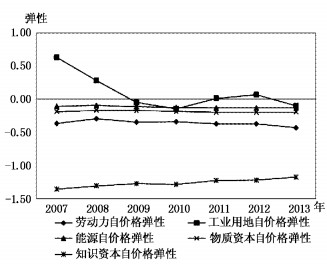图 4 工业用地与其他要素的自价格弹性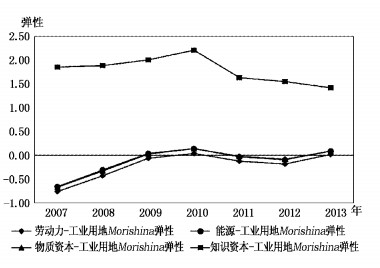图 5 工业用地与其他要素的Morishima替代弹性

(三) 要素相对价格变动的长期技术选择效应

 2007-2013年 工业用地L 劳动力W 能源E 物质资本Kw 知识资本Ke 诱致性技术进步ψiL 全国 -0.001(-0.16) -0.002(-1.01) -0.046***(-5.75) -0.027***(-3.31) 0.011*(1.84) 东部 -0.021***(-3.00) 0.001(0.23) -0.045***(-4.22) -0.076***(-3.91) 0.027*(1.88) 中部 0.013**(2.02) -0.009**(-2.04) -0.015(-0.41) 0.030(-0.93) 0.015(1.12) 西部 0.008(1.24) -0.003(-0.77) -0.048***(-3.34) -0.029**(-2.52) 0.012(1.35) 外生性技术进步BiL 全国 -0.001(-0.58) 0.003(1.63) -0.008**(-2.46) 0.098***(60.91) 0.001(0.63)

  林毅夫, 苏剑. 论我国经济增长方式的转换[J].管理世界,2007(11):5–13.  鲁成军, 周端明. 中国工业部门的能源替代研究——基于对ALLEN替代弹性模型的修正[J].数量经济技术经济研究,2008(5):30–42.  樊茂清, 郑海涛, 孙琳琳, 等. 能源价格、技术变化和信息化投资对部门能源强度的影响[J].世界经济,2012(5):22–45.  王班班, 齐绍洲. 有偏技术进步、要素替代与中国工业能源强度[J].经济研究,2014(2):115–127.  赵爱栋, 马贤磊, 曲福田, 等. 基于资源价值显化视角的中国工业用地市场发育水平及其影响因素[J].资源科学,2016a(2):217–227.  赵爱栋, 马贤磊, 曲福田. 市场化改革能提高中国工业用地利用效率吗?[J].中国人口·资源与环境,2016b(3):118–126.  Acemoglu D, Finkelstein A. Input and technology choices in regulated industries:Evidence from the health care sector[J].Journal of Political Economy,2008,116(5): 837–880.  Esposti R, Pierani P. Price-induced technical progress in Italian agriculture[J].Review of Agricultural and Environmental Studies,2008,89(4): 5–28.  Peeters L, Surry Y. Incorporating price-induced innovation in a symmetric generalised McFadden cost function with several outputs[J].Journal of Productivity Analysis,2000,14(1): 53–70.  RolandG. Transition and economics:Politics, markets and firms[M]. Cambridge: MIT Press, 2000 .  Wing S I. Representing induced technological change in models for climate policy analysis[J].Energy Economics,2006,28(5-6): 539–562.